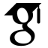Titel: Numerical Algorithms for the Linear-Quadratic Optimal Control of Well-Posed Linear Systems Sonstige Titel: Numerische Algorithmen für die linear-quadratische optimale Steuerung von wohl-definierten linearen Systemen Sprache: Englisch Autor*in: Massoudi, Arash Erscheinungsdatum: 2016 Tag der mündlichen Prüfung: 2017-04-12 Zusammenfassung: In this dissertation we develop two algorithms for solving the linear-quadratic optimal control problem of externally stable well-posed linear systems. The first algorithm is an extension of the Alternating Direction Implicit (ADI) iteration in order to solve the regular linear-quadratic optimal control problem. The algorithm is based on approximating the output map and the input-output map of the well-posed linear system using projections on appropriate subspaces. These projections are determined by the so-called “shift parameters”. We prove that the approximation obtained by this algorithm expresses the optimal cost for a projected optimal control problem. Furthermore, we show that the sequence of approximate solutions obtained by this algorithm is monotonically non-decreasing. Under mild assumptions on the shift parameters, we prove convergence of this sequence to the optimal cost operator (Riccati operator). Later on, we turn our focus to the singular linear-quadratic optimal control problem in the bounded real and positive real case. We show that the ADI iteration can be applied to find approximate solutions of these singular optimal control problems. By assuming finite-dimensionality of the input and output spaces (which is justified in the actual applications), our method provides approximate solutions in low-rank factored form. In order to show convergence, we establish a connection to the projected singular linear-quadratic optimal control problem. As in the regular case, we show that the sequence of approximate solutions is monotonically non-decreasing. If the shift parameters are chosen appropriately, the sequence converges to the optimal cost of the singular optimal control problem in the bounded real and positive real case. The second algorithm is an extension of the Newton-Kleinman iteration to the infinite-dimensional spaces. We propose an extension for solving the regular linear-quadratic optimal control problem subject to regular linear systems which have regular dual systems. We construct a sequence of infinite-time observability Gramians to approximate the Riccati operator. These Gramians have finite rank, if the input and output spaces are finite-dimensional. The feasibility of iterations is shown with the help of an interconnection of the system with its anticausal dual. In addition, we establish a direct connection between the Riccati operator and the sequence of infinite-time observability Gramians. In order to prove monotonicity and convergence of our algorithm, we further assume strong stability of the semigroup and boundedness of the control operator. Moreover, the quadratic rate of convergence of the Newton-Kleinman iterations is proven under the additional assumption of exponentially stability on the semigroup. The presented numerical example suggests that it is even possible to apply our method in case of unbounded control operators. However, the proof of convergence in this case requires more investigations and is left as an open problem. The two algorithms given in this dissertation are developed for the class of well-posed linear systems. If these systems arise from abstract formulation of partial differential equations, then our algorithms allow numerical solutions of the optimal control problems using the approach “optimizing-then-discretizing”. We verify the applicability of our algorithms by applying them to a two-dimensional heat equation with Robin boundary control and boundary integral observation. We present three numerical examples. The first two deal with the regular linear-quadratic optimal control problem. The Riccati operator associated with these examples is nuclear. We find approximations of the Riccati operator once by applying the ADI method and once by employing the Newton-Kleinman iteration. The last example demonstrates the applicability of the ADI method for solving the singular linear-quadratic optimal control problem in the positive real case. A correct choice of shift parameters is crucial for convergence of the ADI method. We propose an effective strategy for choosing the shift parameters based on the stable eigenvalues of the even matrix pencil. In all the examples, monotonicity and convergence of the approximate solutions are illustrated by observing the nuclear norm of the approximations at each iteration. URL: https://ediss.sub.uni-hamburg.de/handle/ediss/7617 URN: urn:nbn:de:gbv:18-90551 Dokumenttyp: Dissertation Betreuer*in: Reis, Timo (Prof. Dr.) Enthalten in den Sammlungen: Elektronische Dissertationen und Habilitationen

###### Dateien zu dieser Ressource:
Datei Beschreibung GrößeFormat
Dissertation.pdf2.67 MBAdobe PDF

Diese Publikation steht in elektronischer Form im Internet bereit und kann gelesen werden. Über den freien Zugang hinaus wurden durch die Urheberin / den Urheber keine weiteren Rechte eingeräumt. Nutzungshandlungen (wie zum Beispiel der Download, das Bearbeiten, das Weiterverbreiten) sind daher nur im Rahmen der gesetzlichen Erlaubnisse des Urheberrechtsgesetzes (UrhG) erlaubt. Dies gilt für die Publikation sowie für ihre einzelnen Bestandteile, soweit nichts Anderes ausgewiesen ist.

Info

#### Seitenansichten

52
Letzte Woche
Letzten Monat
geprüft am 14.04.2021

#### Download(s)

17
Letzte Woche
Letzten Monat
geprüft am 14.04.2021
WerkzeugePrüfe